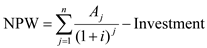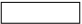# Quiz 9: Managing Research and Development

Net Present Worth (NPW) is calculated using the following formula.Where, i represents the annual rate of return A j is the return from the investment n is the number of years Investment is the amount of money spent As per the question specifications, i = 0.15, A j = \$60,000, n = 2, Investment = \$100,000 Therefore,The NPW of the proposal is -\$2,457.47 This means that the company would be better off without this investment and therefore the proposal should not be funded.